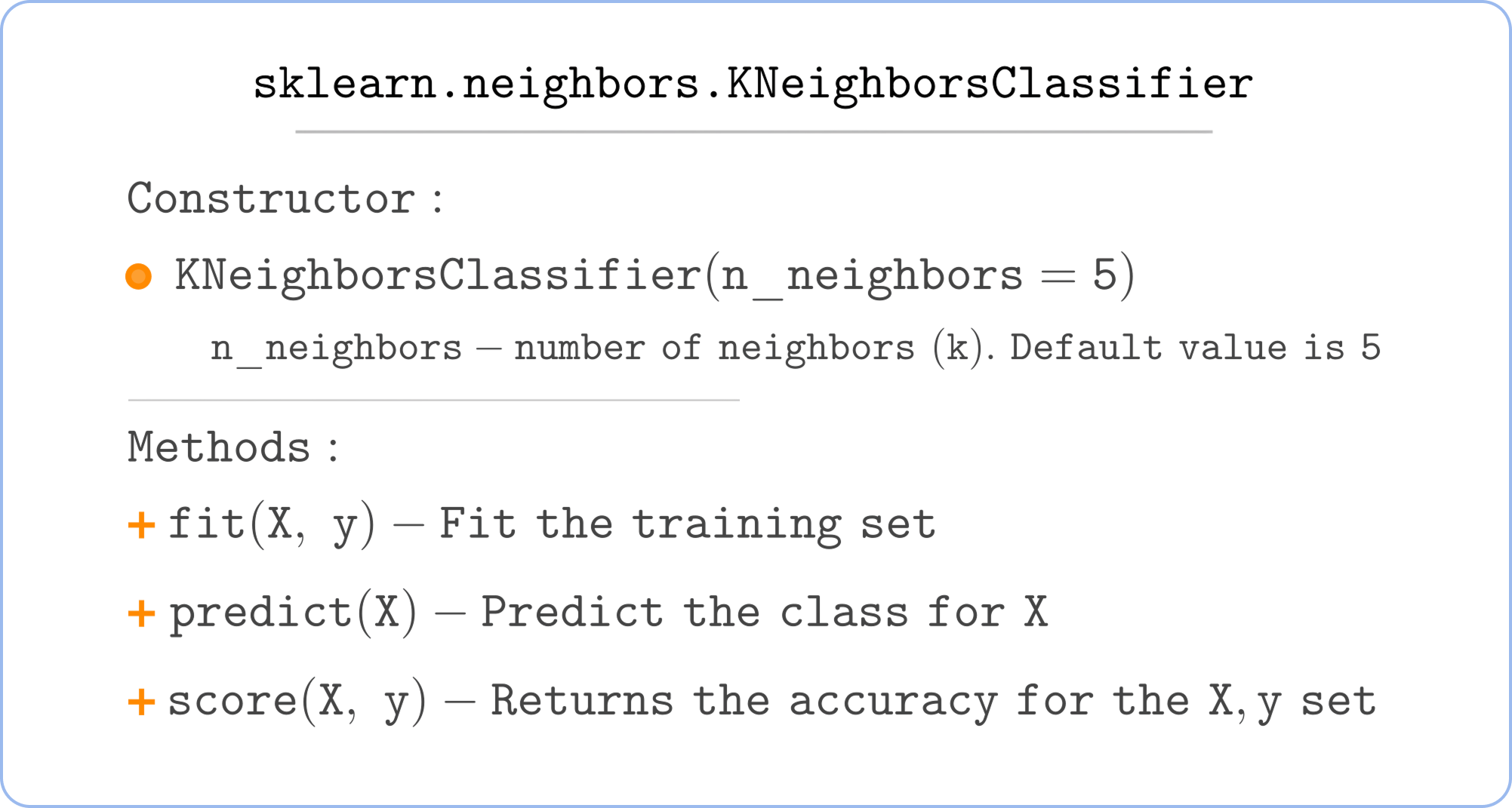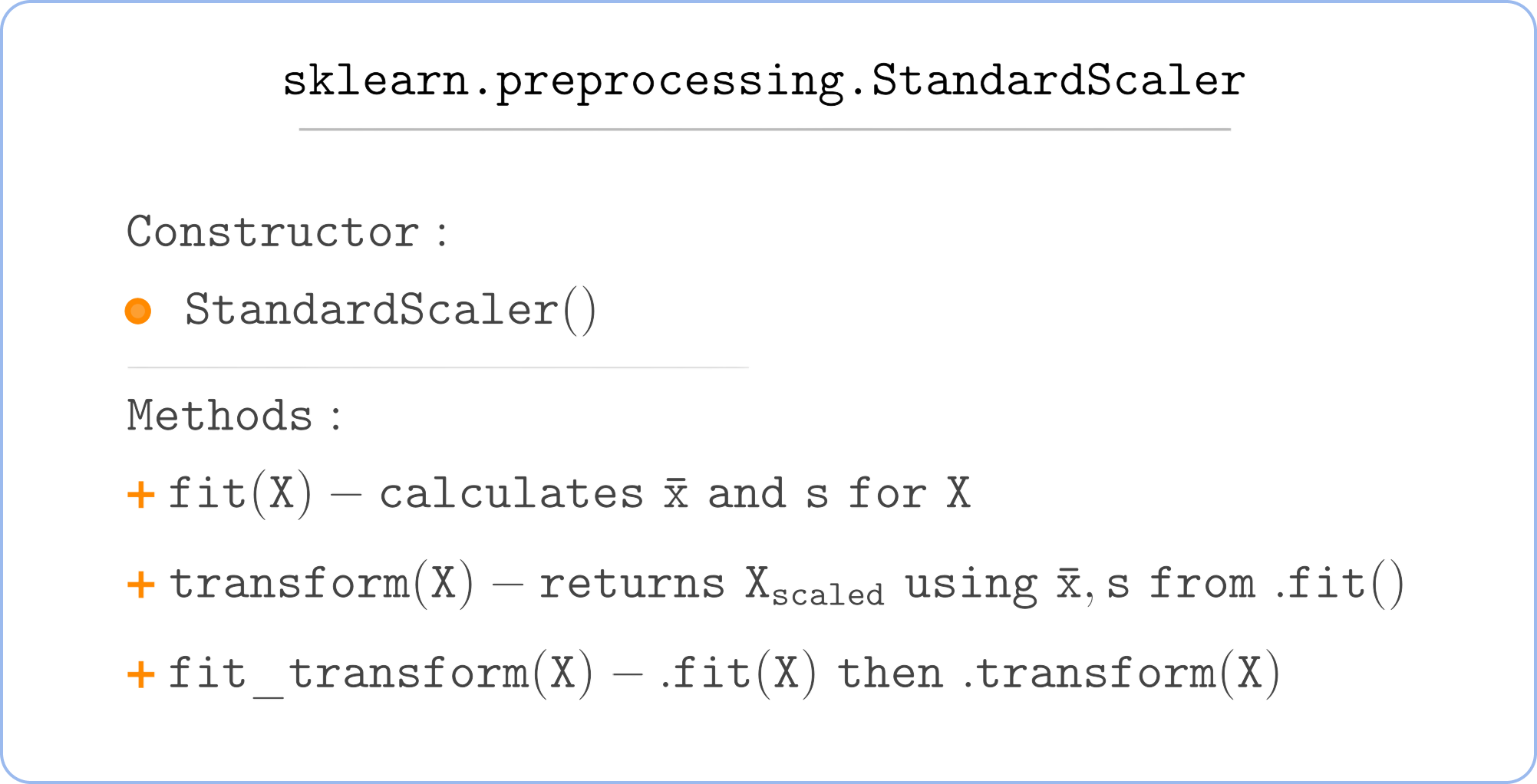Course Content

Classification with Python

##Multi-Class Classification

Multi-class classification with k-NN is as easy as binary classification. We just pick the class that prevails in the neighborhood.

The `KNeighborsClassifier` automatically performs a multi-class classification if `y` has more than two features, so you do not need to change anything. The only thing that changes is the `y` variable fed to the `.fit()` method.

Now you will perform a Multi-class classification with k-NN.
Consider the following dataset:It is the same as in the previous chapter's example, but now the target can take three values:

• 0 – "Hated it" (rating is less than 3/5);
• 1 – "Meh" (rating between 3/5 and 4/5);
• 2 – "Liked it" (rating is 4/5 or higher).

Let's move to classification! Well, wait, here is the reminder of the classes you will use.And now, let's move to classification!

Perform a classification using the `KNeighborsClassifier` with `n_neighbors` equal to `13`.

1. Import the `KNeighborsClassifier`.
2. Use the appropriate class to scale the data.
3. Scale the data using `.fit_transform()` for training data and `.transform()` for new instances.
4. Create the `KNeighborsClassifier` object and feed `X_scaled` and `y` to it.
5. Predict the classes for new instances (`X_new_scaled`)

Once you've completed this task, click the button below the code to check your solution.

Everything was clear?

Section 1. Chapter 5